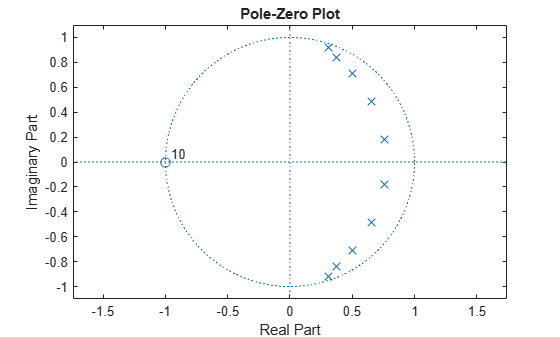# isstable

Verify that discrete-time filter System object is stable

## Syntax

``flag = isstable(sysobj)``
``flag = isstable(sysobj,'Arithmetic',arithType)``

## Description

example

````flag = isstable(sysobj)` returns `true` if filter System object™ is stable. The function returns `false` if filter System object is not stable.```
````flag = isstable(sysobj,'Arithmetic',arithType)` analyzes the filter System object based on the arithmetic specified in the `arithType` input.For more input options, see `isstable` in Signal Processing Toolbox™.```

## Examples

collapse all

Design a Chebyshev Type I IIR filter and determine if the filter has minimum phase and is stable.

Using the `fdesign.lowpass` and `design` functions, design a Chebyshev Type I IIR filter with a passband ripple of 0.5 dB and a 3 dB cutoff frequency at 9600 Hz.

```Fs = 48000; % Sampling frequency of input signal d = fdesign.lowpass('N,F3dB,Ap', 10, 9600, .5, Fs); filt = design(d,'cheby1','Systemobject',true)```
```filt = dsp.BiquadFilter with properties: Structure: 'Direct form II' SOSMatrixSource: 'Property' SOSMatrix: [5x6 double] ScaleValues: [6x1 double] InitialConditions: 0 OptimizeUnityScaleValues: true Show all properties ```

Using the `isminphase` function, determine if the filter has minimum phase.

`isminphase(filt)`
```ans = logical 1 ```

Verify the location of poles and zeros of the filter transfer function on the z-plane. By definition, the poles and zeros of the minimum phase filter must be on or inside the unit circle.

`zplane(filt)`All minimum phase filters are stable. To verify if the designed filter is stable, use the `isstable` function.

`isstable(filt)`
```ans = logical 1 ```

## Input Arguments

collapse all

Arithmetic used in the filter analysis, specified as `'double'`, `'single'`, or `'Fixed'`. When the arithmetic input is not specified and the filter System object is unlocked, the analysis tool assumes a double-precision filter. When the arithmetic input is not specified and the System object is locked, the function performs the analysis based on the data type of the locked input.

The `'Fixed'` value applies to filter System objects with fixed-point properties only.

When the `'Arithmetic'` input argument is specified as `'Fixed'` and the filter object has the data type of the coefficients set to `'Same word length as input'`, the arithmetic analysis depends on whether the System object is unlocked or locked.

• unlocked –– The analysis object function cannot determine the coefficients data type. The function assumes that the coefficients data type is signed, has a 16-bit word length, and is auto scaled. The function performs fixed-point analysis based on this assumption.

• locked –– When the input data type is `'double'` or `'single'`, the analysis object function cannot determine the coefficients data type. The function assumes that the data type of the coefficients is signed, has a 16-bit word length, and is auto scaled. The function performs fixed-point analysis based on this assumption.

To check if the System object is locked or unlocked, use the `isLocked` function.

When the arithmetic input is specified as `'Fixed'` and the filter object has the data type of the coefficients set to a custom numeric type, the object function performs fixed-point analysis based on the custom numeric data type.

## Output Arguments

collapse all

Flag to determine if the filter is stable, returned as a logical:

• `1` –– Filter is stable.

• `0` –– Filter is not stable.

Data Types: `logical`

## Version History

Introduced in R2013a